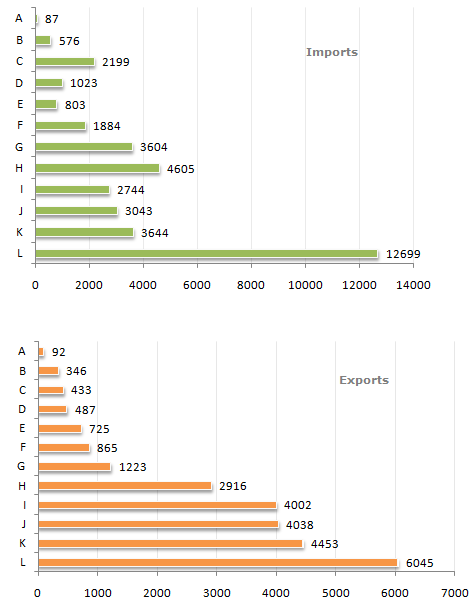Data Interpretation - Bar Charts

Study the following bar charts and answer the questions.

Foreign Trade (Imports and Exports) by countries for the year (1993 - 1994)1.

The ratio of the maximum exports to the minimum imports was closest to ?

 A. 64 B. 69 C. 74 D. 79

Explanation:

The value of maximum exports = 6045.

The value of minimum imports = 87.

Therefore, the required ratio (6045/87) = 69.48 = 69 (approximately).

2.

How many countries exhibited a trade surplus ?

 A. 5 B. 4 C. 3 D. 6

Explanation:

Out of a total of 12 countries, 8 showed a deficit while 4 showed a surplus.

3.

The total trade deficit/surplus for all the countries put together was ?

 A. 11286 surplus B. 11286 deficit C. 10286 deficit D. None of these

Explanation:

Sum of exports - Sum of imports = deficit(11286).

4.

The highest trade deficit was shown by which country ?

 A. C B. G C. H D. L

Explanation:

Visually its clear that L has the highest trade deficit.

5.

The ratio of Exports to Imports was highest for which country ?

 A. A B. I C. J D. K